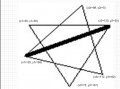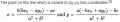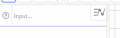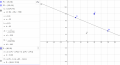Reflection of a Point Over a Line

zulfi100

Joined Jun 7, 2012
495
Hi,

I have a figure containing a triangle and a rotated triangle superimposed at the centroid. I have joined two points of the resultant overlapped image created from the original and the rotated triangles. Now I want to do a reflection over this oblique line. I searched the internet but i cant find any equation. Eq. are provided for reflecting over x-axis, y-axis , over the line x=2 & over the line x=y but no equations are shown for reflecting object over an oblique line. One site gave the method to find the eq of line which was used to reflect the triangle but it didnt give any example for reverse case. Its link was:

http://www.rcboe.org/cms/lib010/GA01903614/Centricity/Domain/1030/Reflections.pdf
I have attached the figure. I want to reflect portions of the two triangles over the dark fat line.
Some body please guide me.Zulfi.

MrAl

Joined Jun 17, 2014
6,410
Hello,

Simply, it looks like if you were to rotate the dark line and get rid of the offset and used that info to rotate every other point on the graph too, then any point could now be reflected about that dark line by simply calculating the negative of that points y value. If you then rotate that new point back and add the offset back, you get that point rotated about the original line. You could analyze this process and come up with a formula.

•zulfi100

zulfi100

Joined Jun 7, 2012
495
Hi,
Thanks for your response. I have to just reflect only four points and then join them with the end-point of the fat line by drawing lines.
Simply, it looks like if you were to rotate the dark line and get rid of the offset and used that info to rotate every other point on the graph too, then any point could now be reflected about that dark line by simply calculating the negative of that points y value.
Can you please show this mathematically? Why using rotation for doing reflection? Why not perform the reflection directly? Do you mean rotation about an arbitrary axis?

Zulfi.

Last edited:

MrChips

Joined Oct 2, 2009
19,157
2-D and 3-D graphics take advantage of matrix operations. The basic operations are addition and multiplication on matrices.
Do you know how to do this?
MrAl has laid out the correct procedure.

Translation is accomplished by matrix addition.
Rotation is accomplished by matix multiplication.

Step 1 - Translate (x1r, y1r) to (0,0) by adding (-x1r, -y1r)
Step 2 - Rotate about (0,0)
Step 3 - Form the reflection by multiplying with (1, -1)
Step 4 - Do reverse rotation
Step 5 - Do reverse translation

Tesla23

Joined May 10, 2009
374
I think this is easiest with vectors:

Representing a point P by a vector P from the origin

a line L with unit normal N and passing through a point Po is all points P:
(P - Po).N = 0

where . is the vector dot product

the vector from any point Px to the foot of it's perpendicular to the line L is
(Px - Po).N N

where we have used the fact that N is a unit normal i.e. |N| = 1

so the reflection of Px in the line L is

Px - 2 (Px - Po).N N

Last edited:

zulfi100

Joined Jun 7, 2012
495
Hi,

Thanks for showing interest in my problem.
Mr. Chips
Step 2 - Rotate about (0,0)
What would be the angle of rotation?
Mr. Tesla:
Representing a point P by a vector P from the origin

a line L with unit normal N and passing through a point Po is all points P:
(P - Po).N = 0
Sorry I cant understand this. If you have time, kindly transform the symbols P , vector P, line L, P0 and N according to my diagram.

Zulfi.

MrChips

Joined Oct 2, 2009
19,157
Your line is (x1r, y1r) - (x2, y2).
You want to make this line horizontal by rotating about (x1r, y1r) as the origin.
Do you know how to calculate the slope of this line?

Tesla23

Joined May 10, 2009
374
write the line as
a*(x - x0) + b*(y - y0) = 0 with a*a + b*b = 1, then the unit normal is n = ai + bj and Po = (x0, y0) is a point on the line

So for a general point Px = (xp, yp)

write d = (Px - Po).n = (xp - x0)*a + (yp - y0)*b

and so the reflection of Px in the line has coordinates (xp - 2*d*a, yp - 2*d*b)

You should check this, it's possible I've messed up a sign, but the method's sound.

zulfi100

Joined Jun 7, 2012
495
Hi,

Thanks Mr. Chips:
Slope would be:

m= (y2-y1r)/(x2-x1r)
From one site I got this formula:
Math.atan2(yDiff, xDiff)*180.0/Math.PI;

where atan2 a java function
Thanks. I would try this.
but its confusing.

Hi Mr. Tesla

You mean i have to find a point on the line nearest to a given point. I found the formula:I would try and reply you.
Thanks.

Zulfi.

MrAl

Joined Jun 17, 2014
6,410
Hi,

You could also try doing it outright using variables x,y, and angle A where A is the original angle of the line.
If you rotate x and y using the rotation equations and angle -A to the point xn,yn, then take the negative of yn as -yn, then rotate xn and -yn by angle A, the result is a pair of convenient double angle formulas that are in the same basic form as the rotation equations themselves except for signs and of course the double angles. These two formulas rotate the point x,y about the line with original angle A.
Of course you'll have to add the offset to this procedure.

Last edited:

MrChips

Joined Oct 2, 2009
19,157
You now have all the steps to do this. Since this is homework, I will leave it up to you to proceed.

Step 1 - Translate (x1r, y1r) to (0,0) by adding (-x1r, -y1r) to the given point (x,y)
Step 2 - Rotate about (0,0). Angle θ = atan(slope)
Step 3 - Form the reflection by multiplying with (1, -1)
Step 4 - Do reverse rotation
Step 5 - Do reverse translation

You now have all the information to do this for a single point. If you wanted to do this for a large collection of points you would do this using matrix arithmetic.

•zulfi100

Tesla23

Joined May 10, 2009
374
Hi Mr. Tesla

You mean i have to find a point on the line nearest to a given point. I found the formula:
View attachment 125285
I would try and reply you.
Thanks.

Zulfi.
No, the reflection of (xp, yp) is (xp - 2*d*a, yp - 2*d*b), you should try to understand the method if you are using it. All you need is the equation of the line and the definition of d = (xp - x0)*a + (yp - y0)*b. (x0, y0) is any point on the line (intercept is fine).

The translation/rotation/reflection method will work as well and use that if you understand it, but this will give the same answer and I think is simpler.

Last edited:

MrAl

Joined Jun 17, 2014
6,410
Hello again,

Here are the two regular rotation equations for a single rotation:
xn:cos(a)*x-sin(a)*y;
yn:sin(a)*x+cos(a)*y;

The point (x,y) is rotated by angle 'a' to the point (xn,yn).

Here are the solutions to the reflected point i found:
X=cos(2*a)*x+sin(2*a)*y-sin(2*a)*b
Y=sin(2*a)*x-cos(2*a)*y+cos(2*a)*b+b

where the point (x,y) is reflected about the line with angle 'a' and y intercept 'b', and (X,Y) is the resulting point.
Note the similarities to the rotation equations, but did i make a mistake perhaps in one of the signs or even the entire result? That's up to you to determine. You should try to prove that result and see if you can or if you can disprove it.

Tesla23

Joined May 10, 2009
374
You mean i have to find a point on the line nearest to a given point. I found the formula:Of course, if this formula is correct (I'm not saying it isn't, but I haven't checked it), then it will give you the answer as well, as the closest point on the line to the point P is the foot of the perpendicular, call it F. If you have found F then you can find the reflected point directly by going from P to F and going as far again in the same direction. So if P is (x0, y0), and if F (x0 + dx, y0 + dy), then the reflection point R is (x0 + 2*dx, y0 + 2*dy). In other words, as F is the midpoint of P and R, if you know P and F you can find R. This is really the method of the vector solution I've been explaining.

Last edited:

MrChips

Joined Oct 2, 2009
19,157
Make sure you get the sign of the angle correct.

zulfi100

Joined Jun 7, 2012
495
Hi,
I would should show you my JAVA code:
Code:
//reflecting points (x1, y1), (x2r, y2r), (x3, y3) & (x3r, y3r) about the line
//having end points (x1r, y1r)-(x2,y2)
//Step 1 - Translate (x1r, y1r) to (0,0) by adding (-x1r, -y1r) to the given point (x,y)
Double x1T= x1-x1r;
Double y1T= y1-y1r;
Double x2rT= x2r-x1r;
Double y2rT= y2r-y1r;
Double x3T = x3-x1r;
Double y3T = y3-y1r;
Double x3rT = x3r-x1r;
Double y3rT = y3r -y1r;

//Step 2 - Rotate about (0,0). Angle ? = atan(slope)
Double slope = (y2-y1r)/(x2-x1r);
Double Angle = Math.atan(slope);
Double x1Tr = x1T*Math.cos(Angle * Math.PI/180.0) - y1T*Math.sin(Angle * Math.PI/180.0);
Double y1Tr = x1T*Math.sin(Angle * Math.PI/180.0) + y1T*Math.cos(Angle * Math.PI/180.0);
Double x2rTr = x2rT*Math.cos(Angle * Math.PI/180.0) - y2rT*Math.sin(Angle * Math.PI/180.0);
Double y2rTr = x2rT*Math.sin(Angle * Math.PI/180.0) + y2rT*Math.cos(Angle * Math.PI/180.0);
Double x3Tr =  x3T*Math.cos(Angle * Math.PI/180.0) - y3T*Math.sin(Angle * Math.PI/180.0);
Double y3Tr =  x3T*Math.sin(Angle * Math.PI/180.0) + y3T*Math.cos(Angle * Math.PI/180.0);
Double x3rTr = x3rT*Math.cos(Angle * Math.PI/180.0) - y3rT*Math.sin(Angle * Math.PI/180.0);
Double y3rTr = x3rT*Math.sin(Angle * Math.PI/180.0) + y3rT*Math.cos(Angle * Math.PI/180.0);

//Step 3 - Form the reflection by multiplying with (1, -1)
Double x1Tr_ref=x1Tr;
Double y1Tr_ref=-y1Tr;
Double x2rTr_ref=x2rTr;
Double y2rTr_ref=-y2rTr;
Double x3Tr_ref=x3Tr;
Double y3Tr_ref=-y3Tr;
Double x3rTr_ref=x3rTr;
Double y3rTr_ref=-y3rTr;

//Step 4 - Do reverse rotation: tried with -Angle also
Double x1T_ref= x1Tr_ref*Math.cos(Angle * Math.PI/180.0) - y1Tr_ref*Math.sin(Angle * Math.PI/180.0);
Double y1T_ref= x1Tr_ref*Math.sin(Angle * Math.PI/180.0) + y1Tr_ref*Math.cos(Angle * Math.PI/180.0);
Double x2rT_ref = x2rTr_ref*Math.cos(Angle * Math.PI/180.0) - y2rTr_ref*Math.sin(Angle * Math.PI/180.0);
Double y2rT_ref = x2rTr_ref*Math.sin(Angle * Math.PI/180.0) + y2rTr_ref*Math.cos(Angle * Math.PI/180.0);
Double x3T_ref= x3Tr_ref*Math.cos(Angle * Math.PI/180.0) - y3Tr_ref*Math.sin(Angle * Math.PI/180.0);
Double y3T_ref= x3Tr_ref*Math.sin(Angle * Math.PI/180.0) + y3Tr_ref*Math.cos(Angle * Math.PI/180.0);
Double x3rT_ref = x3rTr_ref*Math.cos(Angle * Math.PI/180.0) - y3rTr_ref*Math.sin(Angle * Math.PI/180.0);
Double y3rT_ref = x3rTr_ref*Math.sin(Angle * Math.PI/180.0) + y3rTr_ref*Math.cos(Angle * Math.PI/180.0);

//Step 5 - Do reverse translation
Double x1_ref= x1T_ref + x1r;
Double y1_ref= y1T_ref + y1r;
Double x2r_ref = x2rT_ref + x1r;
Double y2r_ref = y2rT_ref + y1r;
Double x3_ref= x3T_ref + x1r;
Double y3_ref= y3T_ref + y1r;
Double x3r_ref = x3rT_ref +x1r;
Double y3r_ref = y3rT_ref +y1r;

}
I checked the input values inside the method & values are correct:
x1=30.0 y1=30.0
x3=75.0 y3=107.94228634059948
x2r=89.14213562373095 y2r=5.50252531694
x3r=112.43584968295782 y3r=92.43584968295781

After reflection, I am getting following output values
ix1_ref=30
iy1_ref=108
ix2r_ref=90
iy2r_ref=131
ix3_ref=74
iy3_ref=29
ix3r_ref=112
iy3r_ref=44

Some body please check what's the problem with this code.
I would try with other method.
Zulfi.

Last edited:

Tesla23

Joined May 10, 2009
374
I was bored and tried this in Geogebra (https://www.geogebra.org/apps/) where you can setup an interactive geometry online.

Try this https://www.geogebra.org/m/GsX6VnEw and move P around.

This was created by going into GeoGebra Math Apps, and entering into the box:the following (you need to rescale a bit using the mouse wheel and some panning):

P1=(25,69)
P2=(120,30)
L1=Line[P1,P2]
a:=x(L1)
b:=y(L1)
a1=a/sqrt(a*a+b*b)
b1=b/sqrt(a*a+b*b)
P=(80,80)
d:=a1*(x(P)-x(P1)) + b1*(y(P)-y(P1))
R=(x(P)-2*d*a1, y(P)-2*d*b1)

you will get:and if you click on the point P with the mouse, you can drag it around and see the reflection point behave accordingly. It's really neat. You can even drag P1 and P2, changing the line etc..

Last edited:
•zulfi100

MrChips

Joined Oct 2, 2009
19,157
Hi,
I would should show you my JAVA code:
Code:
//reflecting points (x1, y1), (x2r, y2r), (x3, y3) & (x3r, y3r) about the line
//having end points (x1r, y1r)-(x2,y2)
//Step 1 - Translate (x1r, y1r) to (0,0) by adding (-x1r, -y1r) to the given point (x,y)
Double x1T= x1-x1r;
Double y1T= y1-y1r;
Double x2rT= x2r-x1r;
Double y2rT= y2r-y1r;
Double x3T = x3-x1r;
Double y3T = y3-y1r;
Double x3rT = x3r-x1r;
Double y3rT = y3r -y1r;

//Step 2 - Rotate about (0,0). Angle ? = atan(slope)
Double slope = (y2-y1r)/(x2-x1r);
Double Angle = Math.atan(slope);
Double x1Tr = x1T*Math.cos(Angle * Math.PI/180.0) - y1T*Math.sin(Angle * Math.PI/180.0);
Double y1Tr = x1T*Math.sin(Angle * Math.PI/180.0) + y1T*Math.cos(Angle * Math.PI/180.0);
Double x2rTr = x2rT*Math.cos(Angle * Math.PI/180.0) - y2rT*Math.sin(Angle * Math.PI/180.0);
Double y2rTr = x2rT*Math.sin(Angle * Math.PI/180.0) + y2rT*Math.cos(Angle * Math.PI/180.0);
Double x3Tr =  x3T*Math.cos(Angle * Math.PI/180.0) - y3T*Math.sin(Angle * Math.PI/180.0);
Double y3Tr =  x3T*Math.sin(Angle * Math.PI/180.0) + y3T*Math.cos(Angle * Math.PI/180.0);
Double x3rTr = x3rT*Math.cos(Angle * Math.PI/180.0) - y3rT*Math.sin(Angle * Math.PI/180.0);
Double y3rTr = x3rT*Math.sin(Angle * Math.PI/180.0) + y3rT*Math.cos(Angle * Math.PI/180.0);

//Step 3 - Form the reflection by multiplying with (1, -1)
Double x1Tr_ref=x1Tr;
Double y1Tr_ref=-y1Tr;
Double x2rTr_ref=x2rTr;
Double y2rTr_ref=-y2rTr;
Double x3Tr_ref=x3Tr;
Double y3Tr_ref=-y3Tr;
Double x3rTr_ref=x3rTr;
Double y3rTr_ref=-y3rTr;

//Step 4 - Do reverse rotation: tried with -Angle also
Double x1T_ref= x1Tr_ref*Math.cos(Angle * Math.PI/180.0) - y1Tr_ref*Math.sin(Angle * Math.PI/180.0);
Double y1T_ref= x1Tr_ref*Math.sin(Angle * Math.PI/180.0) + y1Tr_ref*Math.cos(Angle * Math.PI/180.0);
Double x2rT_ref = x2rTr_ref*Math.cos(Angle * Math.PI/180.0) - y2rTr_ref*Math.sin(Angle * Math.PI/180.0);
Double y2rT_ref = x2rTr_ref*Math.sin(Angle * Math.PI/180.0) + y2rTr_ref*Math.cos(Angle * Math.PI/180.0);
Double x3T_ref= x3Tr_ref*Math.cos(Angle * Math.PI/180.0) - y3Tr_ref*Math.sin(Angle * Math.PI/180.0);
Double y3T_ref= x3Tr_ref*Math.sin(Angle * Math.PI/180.0) + y3Tr_ref*Math.cos(Angle * Math.PI/180.0);
Double x3rT_ref = x3rTr_ref*Math.cos(Angle * Math.PI/180.0) - y3rTr_ref*Math.sin(Angle * Math.PI/180.0);
Double y3rT_ref = x3rTr_ref*Math.sin(Angle * Math.PI/180.0) + y3rTr_ref*Math.cos(Angle * Math.PI/180.0);

//Step 5 - Do reverse translation
Double x1_ref= x1T_ref + x1r;
Double y1_ref= y1T_ref + y1r;
Double x2r_ref = x2rT_ref + x1r;
Double y2r_ref = y2rT_ref + y1r;
Double x3_ref= x3T_ref + x1r;
Double y3_ref= y3T_ref + y1r;
Double x3r_ref = x3rT_ref +x1r;
Double y3r_ref = y3rT_ref +y1r;

}
I checked the input values inside the method & values are correct:
x1=30.0 y1=30.0
x3=75.0 y3=107.94228634059948
x2r=89.14213562373095 y2r=5.50252531694
x3r=112.43584968295782 y3r=92.43584968295781

After reflection, I am getting following output values
ix1_ref=30
iy1_ref=108
ix2r_ref=90
iy2r_ref=131
ix3_ref=74
iy3_ref=29
ix3r_ref=112
iy3r_ref=44

Some body please check what's the problem with this code.
I would try with other method.
Zulfi.
I see two mistakes.
The angle to rotate is -atan(slope).